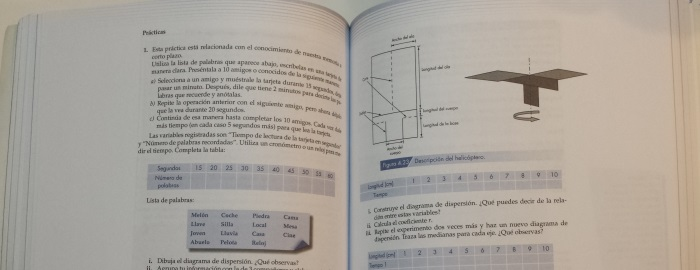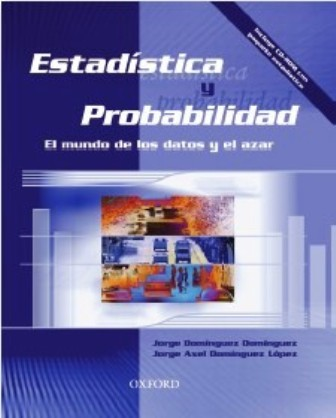## Statistics and Probability: The world of data and random### Statistics and Probability: The world of data and random

#### Domínguez Domínguez, Jorge and Domínguez López, Jorge Axel

##### ISBN: 9706138307

This book on Statistics and Probability differs from others of its kind for two fundamental aspects:

It seeks to illustrate how the two subjects are related to the different areas of human knowledge (economics, medicine, engineering, etc.) and with everyday life. To show that interaction, the authors use an inductive method of exposure, i.e., the topics are explained based on solving real and concrete problems.

It includes a CD which contains a novel, comprehensive and visually appealing statistical package, intended to help students develop different types of graphs, obtaining several measurements and explore practical applications of probability and statistics.

Moreover, the book consists of eight units that are developed in clear and rigorous manner, the topics defined in various syllabus of probability and statistics courses at the High School level. Special attention was paid to the didactic structure of the text. Therefore, each topic begins with a goal, then, problems to be solved step by step are presented; at the end of the solution, an interpretation of results is given, highlighting the importance of statistics and probability in the affairs of our daily life. It also includes numerous solved examples and a diverse number of activities and exercises at the end of each topic and unit. Also at the end of each unit, a summary of the most important concepts is presented. For its innovative approach this book will be very helpful to the student to achieve a greater understanding of the role of statistics and probability, which will result in a better use of these mathematical disciplines.